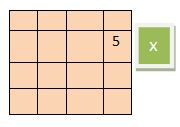# Explore hunderds of solved example topic wise.

## Variables

### Variable

1. Variable is an entity that can change during the program execution.
2. In a more practical sense variable is the name of the memory location where data is stored.3. The given figure will help you to understand the concept. The figure tells, the concept of what happens when a variable is created?
4. Whenever a variable is created in memory a random space is reserved and named with your given label. Here the label is x assign the given value. Here the value is 5.
5. Once a variable is created it is accessed by using the variable name and changed. But remember if you change the value the memory place remains the same and value is changed.
6. The variable name should be meaningful so easy to remember.

#### Type of Variable

If a variable has integer value then the variable is called integer type variable and if the variable contains the float value the variable is considered as float value and same for character and other types of values.

So it is clear variable type is dependent on the type of the constant which is going to store in that variable. As there will be as many types of variables is possible as the number of constant.

#### Variable Declaration

In C every variable must be declared before it is used. The variable declaration statement is called type declaration instruction. Following example some variable declaration.

`int x;float y;char z;`

#### General Syntax

datatype variable_name;

Each variable declaration has two parts. The first part is the data type that is defined by keywords int, float, char and the second part is the name of the variable.

The type of variable can be decided by relating them to the real world. For example, age should be the type of int, price of the type float.